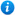* Watermarks do not appear on purchased resources

Preview resource: Click slides to enlarge

# Division - Year 6

Division - Year 6

Year 6 maths programme of study - Number (addition, subtraction, multiplication and division):

• divide numbers up to 4 digits by a two-digit number using the formal written method of long division, interpret remainders as whole number remainders, fractions, or by rounding, as appropriate for the context
• use their knowledge of the order of operations to carry out calculations involving the four operations
• solve problems involving division

This Year 6 maths teaching resource includes a PowerPoint and differentiated worksheets for independent learning, to guide your class through long division to solve calculations and multi-step problems as per the curriculum objectives listed above. It will help the children understand how to solve division problems in context, along with developing their understanding of long division. By exploring division, the resource helps children learn which operation to use and why. The worksheets contain varied fluency, reasoning and problem solving to reinforce and consolidate the children's learning. The resource contains five lessons, which support the White Rose Small Steps guidance, and are designed to support a mastery approach to teaching maths.

Content includes:

1. Lesson 1: Division using factors and 1 accompanying worksheet with answers
2. Lesson 2: Dividing three-digit numbers by two-digit numbers with no remainders and 1 accompanying worksheet with answers
3. Lesson 3: Dividing three and four-digit numbers by two-digit numbers with remainders and 1 accompanying worksheet with answers
4. lesson 4: Dividing four-digit numbers by two-digit numbers with remainders and 1 accompanying worksheet with answers
5. Lesson 5: Consolidation of skills learned so far with challenge cards

'Division - Year 6' is completely editable giving teachers the freedom to adapt the resource to suit their individual teaching needs.

Click on the images from the PowerPoint presentation to view the resource in more detail.

Our Price : £5.99 / 6 Credits## Get this resource as part of a bundle and save up to 82%## Year 6 Home Learning Pack - Maths and English

£14.99

By continuing to use the site, you agree to the use of cookies. You can change this and find out more by following this link